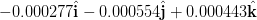# Force between 2 point charges in VECTOR format (x i + y j + z k)

• nataelp
Coulomb's law:$$\vec F = k\frac{q_1q_2}{r^2} \hat r$$where ##\vec F## is the force of ##q_2## on ##q_1## and ##\hat r## is a unit vector from ##q_2## to ##q_1##. And ##r## is the distance between the two charges.How can I calculate a unit vector for force without its components though? Or am I supposed to calculate a unit vector for the distance and then multiply by that?

#### nataelp

Homework Statement
Point charges
q1 = 4.0 µC and q2 = 4.0 µCare located at r1 = (4.0î − 5.0ĵ + 2.0k) mand r2 = (9.0î + 5.0ĵ − 6.0k)m What is the force (in N) of q2 on q1? (Express your answer in vector form.)
Relevant Equations
F = k*q1q1/r^2
I tried using the distance between r2 and r1 and plugging them into the equation for i, j, k. >>
So for the force in the x direction it was k*(4E-6*4E-6)/(4-9)^2. The answer I got was wrong according to webassign. Can someone please tell me what I am missing?

And to be clear it was not the direction of the vector that was wrong, I can see that the magnitude is completely off, but the direction was correct.

nataelp said:
And to be clear it was not the direction of the vector that was wrong, I can see that the magnitude is completely off, but the direction was correct.
What magnitude did you get?

I got (-0.0058, -0.00144, 0.00225)

nataelp said:
I got (-0.0058, -0.00144, 0.00225)
What's the vector from ##q_2## to ##q_1##?

It should be 13.72 using the Pythagorean theorem.

nataelp said:
It should be 13.72 using the Pythagorean theorem.
That is the magnitude of that vector

malawi_glenn said:
That is the magnitude of that vector
If you mean in the vector form, it would be <-5^2, -10^2, 8^2>. And those are the values for r I used to find the force on each axis. The answer WebAssign gave me was this:My problem is I don't know how to get to this answer.

nataelp said:
If you mean in the vector form, it would be <-5^2, -10^2, 8^2>. And those are the values for r I used to find the force on each axis. The answer WebAssign gave me was this:
View attachment 320443
My problem is I don't know how to get to this answer.
I get that answer. How did you try to solve the problem?

•nataelp
So I did this:
For the force along each axis, I used F= k*q1*q2/r^2. I had the charges, and k is a known value, so I just needed the distance r.
For r along the x-axis, for example, I used 4-9 as r because it should be the distance in the x-direction. So I solved it with that value and got my answer for force in the x direction. I repeated it for x, y, and z.
I'm not sure where I am going wrong. And I did change it from microcoloumbs to coloumbs.

nataelp said:
So I did this:
For the force along each axis, I used F= k*q1*q2/r^2. I had the charges, and k is a known value, so I just needed the distance r.
For r along the x-axis, for example, I used 4-9 as r because it should be the distance in the x-direction. So I solved it with that value and got my answer for force in the x direction. I repeated it for x, y, and z.
I'm not sure where I am going wrong. And I did change it from microcoloumbs to coloumbs.
Ah, this is not right. Once you have the magnitude of the force, you just need to calculate a unit vector in the right direction.

•nataelp
So I need to find the magnitude of the vector from q2 to q1 (in m), use that as r, and then to find the force along the i j k directions, I need to calculate a unit vector along those directions? Then multiply by that unit vector, which is each component divided by the magnitude?

nataelp said:
So I need to find the magnitude of the vector from q2 to q1 (in m), use that as r, and then to find the force along the i j k directions, I need to calculate a unit vector along those directions? Then multiply by that unit vector, which is each component divided by the magnitude?
Yes, Coulomb's law is:$$\vec F = k\frac{q_1q_2}{r^2} \hat r$$where ##\vec F## is the force of ##q_2## on ##q_1## and ##\hat r## is a unit vector from ##q_2## to ##q_1##. And ##r## is the distance between the two charges.

•nataelp
How can I calculate a unit vector for force without its components though? Or am I supposed to calculate a unit vector for the distance and then multiply by that? I'm honestly not sure what you mean.

Should the unit vector be <-5, -10, 8>/13.75 (magnitude of distance vector)? Then I multiply "F = k*q1*q2/13.75^2" by that unit vector, and I have my answer?

nataelp said:
For r along the x-axis, for example, I used 4-9 as r because it should be the distance in the x-direction. So I solved it with that value and got my answer for force in the x direction.
No, you don't divide the q1*q2 in each direction by the square of the distance in that direction. You have to divide each by the square of the total distance between the charges.

Last edited:
•nataelp
I have figured out the problem now. Thank you all for your help!

nataelp said:
How can I calculate a unit vector for force without its components though? Or am I supposed to calculate a unit vector for the distance and then multiply by that? I'm honestly not sure what you mean.
I suggest you revise your knowledge of vectors.